# NCERT Solutions for Class 10 Maths Chapter 3 Pair of Linear Equations in Two Variables Ex 3.6

Get Free NCERT Solutions for Class 10 Maths Chapter 3 Ex 3.6 PDF. Pair of Linear Equations in Two Variables Class 10 Maths NCERT Solutions are extremely helpful while doing your homework. Exercise 3.6 Class 10 Maths NCERT Solutions were prepared by Experienced ncert-books.in Teachers. Detailed answers of all the questions in Chapter 3 Maths Class 10 Pair of Linear Equations in Two Variables Exercise 3.6 provided in NCERT TextBook.

Topics and Sub Topics in Class 10 Maths Chapter 3 Pair of Linear Equations in Two Variables:

 Section Name Topic Name 3 Pair of Linear Equations in Two Variables 3.1 Introduction 3.2 Pair Of Linear Equations In Two Variables 3.3 Graphical Method Of Solution Of A Pair Of Linear Equations 3.4 Algebraic Methods Of Solving A Pair Of Linear Equations 3.4.1 Substitution Method 3.4.2 Elimination Method 3.4.3 Cross-Multiplication Method 3.5 Equations Reducible To A Pair Of Linear Equations In Two Variables 3.6 Summary

You can also download the free PDF of  Ex 3.6 Class 10 Pair of Linear Equations in Two Variables NCERT Solutions or save the solution images and take the print out to keep it handy for your exam preparation.

 Board CBSE Textbook NCERT Class Class 10 Subject Maths Chapter Chapter 3 Chapter Name Pair of Linear Equations in Two Variables Exercise Ex 3.6 Number of Questions Solved 2 Category NCERT Solutions

## NCERT Solutions for Class 10 Maths Chapter 3 Pair of Linear Equations in Two Variables Ex 3.6

NCERT Solutions for Class 10 Maths Chapter 3 Pair of Linear Equations in Two Variables Ex 3.6 are part of NCERT Solutions for Class 10 Maths. Here we have given NCERT Solutions for Class 10 Maths Chapter 3 Pair of Linear Equations in Two Variables Exercise 3.6

Question 1.
Solve the following pairs of equations by reducing them to a pair of linear equations:Solution: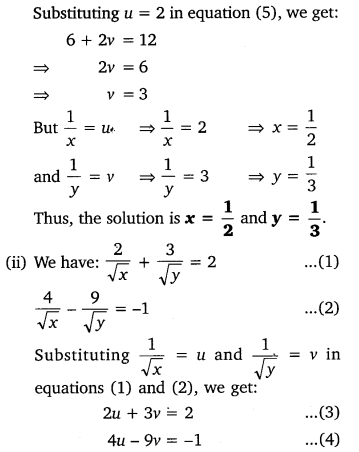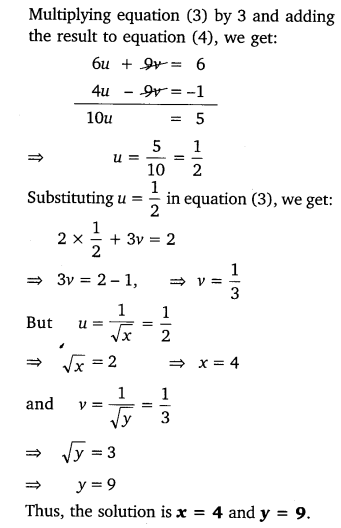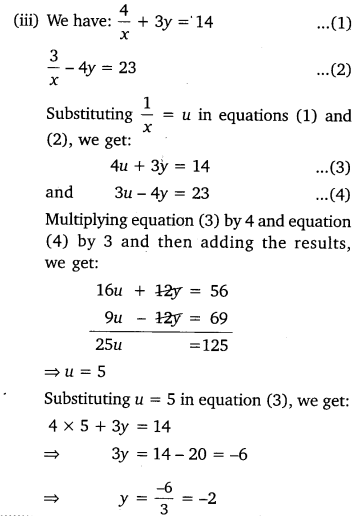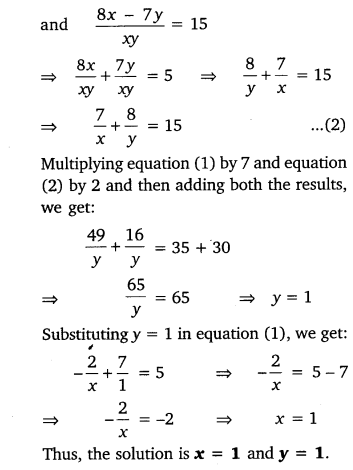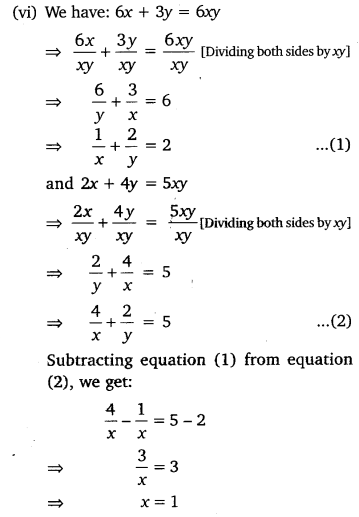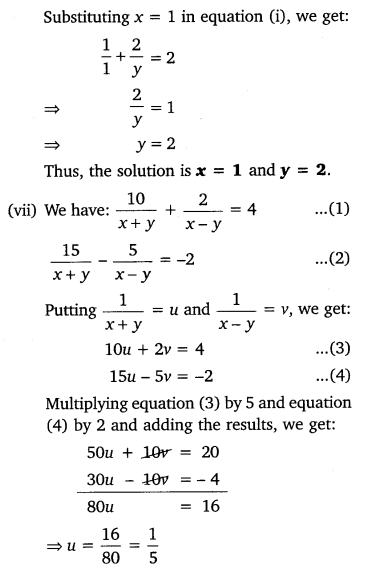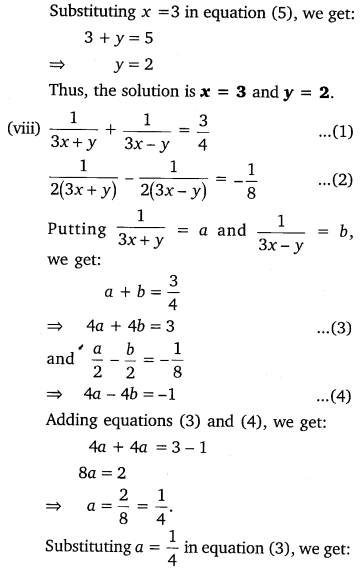Question 2.
Formulate the following problems as a pair of linear equations and hence find their solutions:
(i) Ritu can row downstream 20 km in hours and upstream 4 km in 2 hours. Find her speed of rowing in still water and the speed of the current.
(ii) 2 women and 5 men can together finish an embroidery work in 4 days, while 3 women and 6 men can finish it in 3 days. Find the time taken by 1 woman alone to finish the work, and also that taken by 1 man alone.
(iii) Roohi travels 300 km to her home partly by train and partly by bus. She takes 4 hours if she travels 60 km by train and the remaining by bus. If she travels 100 km by train and the remaining by bus, she takes 10 minutes longer. Find the speed of the train and the bus separately.
Solution: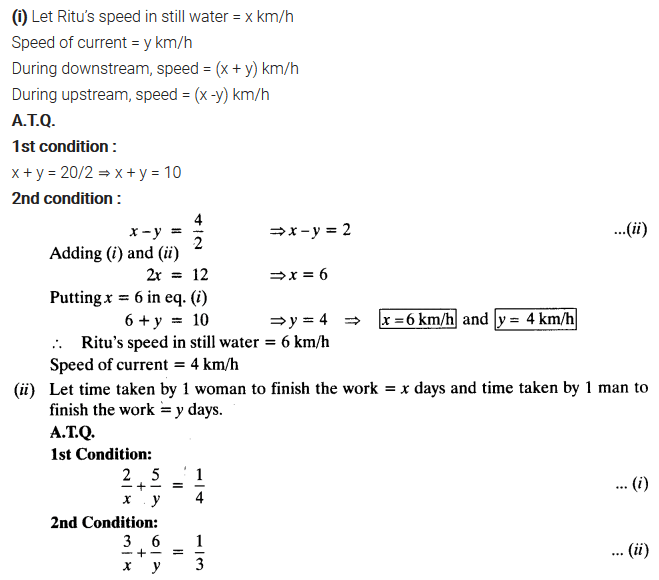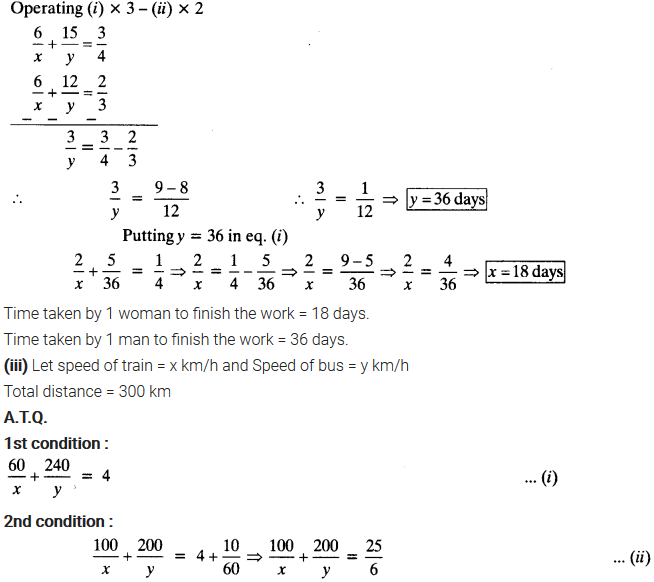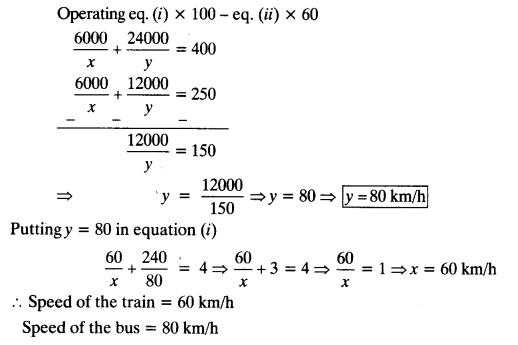+